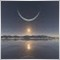# Average Range847

Hi all, I wrote this code to calculate the average range between high and low.

Could you tell me if it's ok or have you got a suggestion on how to improve it?

Thank you

Where Periodo is an external int.

```int start()
{
int limit;
int counted_bars=IndicatorCounted();

//---- last counted bar will be recounted

if(counted_bars>0) counted_bars--;
limit=Bars-counted_bars;

//---- calcolo entry_long
for(int i=0; i<limit; i++)
{
Somma = 0 ;
for(int j=i+1; j<=i+Periodo; j++)
{
Somma += (High[j]-Low[j]) ;
}
// Histogram[i] = 10 ;
Histogram[i] = Somma/Periodo ;
}
return(0);
//---- done```627

Moving Average function also suits for averages

```int start()
{
int limit;
int counted_bars=IndicatorCounted();

//---- last counted bar will be recounted
if(counted_bars<0) return(-1);
if(counted_bars>0) counted_bars--;
limit=Bars-counted_bars;

//---- calcolo entry_long
for(int i=0; i<limit; i++) Histogram[i] = iMA(_Symbol,_Period,Periodo,0,MODE_SMA,PRICE_HIGH,i)- iMA(_Symbol,_Period,Periodo,0,MODE_SMA,PRICE_LOW,i);

return(0);
}
//---- done```